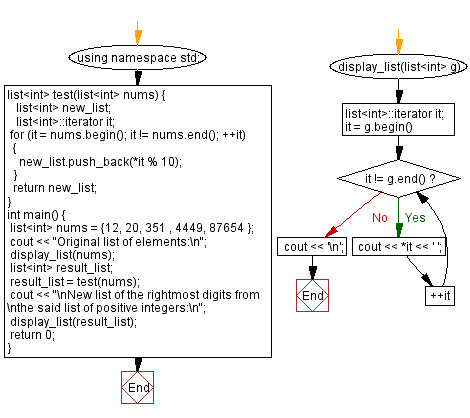﻿ CPP - Create a list of the rightmost digits of positive integers

# C++ Exercises: From a list of positive integers, create a list of their rightmost digits

## C++ Basic Algorithm: Exercise-129 with Solution

Write a C++ program to create a new list of the rightmost digits from a given list of positive integers.

Test Data:
({ 12, 20, 351, 4449}) -> {2, 0, 1, 9}

Sample Solution:

C++ Code :

``````#include <bits/stdc++.h>
#include <list>

using namespace std;

list<int> test(list<int> nums) {
list<int> new_list;
list<int>::iterator it;
for (it = nums.begin(); it != nums.end(); ++it)
{
new_list.push_back(*it % 10);
}

return new_list;
}

display_list(list<int> g)
{
list<int>::iterator it;
for (it = g.begin(); it != g.end(); ++it)
cout << *it << ' ';
cout << '\n';
}

int main() {

list<int> nums = {12, 20, 351 , 4449, 87654 };
cout << "Original list of elements:\n";
display_list(nums);
list<int> result_list;
result_list = test(nums);
cout << "\nNew list of the rightmost digits from \nthe said list of positive integers:\n";
display_list(result_list);
return 0;
}

``````

Sample Output:

```Original list of elements:
12 20 351 4449 87654

New list of the rightmost digits from
the said list of positive integers:
2 0 1 9 4
```

Flowchart:C++ Code Editor:

What is the difficulty level of this exercise?

﻿

## C++ Programming: Tips of the Day

What is the usefulness of `enable_shared_from_this?

It enables you to get a valid shared_ptr instance to this, when all you have is this. Without it, you would have no way of getting a shared_ptr to this, unless you already had one as a member.

```class Y: public enable_shared_from_this
{
public:

shared_ptr f()
{
return shared_from_this();
}
}

int main()
{
shared_ptr p(new Y);
shared_ptr q = p->f();
assert(p == q);
assert(!(p < q || q < p)); // p and q must share ownership
}
```

The method f() returns a valid shared_ptr, even though it had no member instance. Note that you cannot simply do this:

```class Y: public enable_shared_from_this
{
public:

shared_ptr f()
{
return shared_ptr(this);
}
}
```

The shared pointer that this returned will have a different reference count from the "proper" one, and one of them will end up losing and holding a dangling reference when the object is deleted.

Ref : https://bit.ly/3pwVzzz